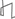Research Abstracts Home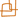CSAIL Digital Archive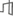Research Activities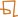CSAIL HomeResearch Abstracts - 2007IntroductionPerception & LearningPhysical, Biological & Social SystemsSystemsTheory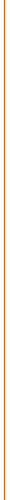### Testing K-Wise and Almost K-Wise Independence

#### N. Alon, Alexandr Andoni, Tali Kaufman, Kevin Matulef, Ronitt Rubinfeld & Ning Xie

##### Results for K-Wise Independence

This work  studies upper and lower bound on the distance of a distribution from the set of k-wise independence distributions. The bounds obtained are a quadratic improvement over similar bounds that were previously obtained by Alon Goldreich and Mansour . We also turn these bounds into an almost optimal testing algorithm that gets samples from a distribution and decides whether the given distribution is k-wise independent or far from any k-wise independent distribution. The complexity of the testing is measured by the number of samples taken from the input distribution.

##### Results for Almost K-Wise Independence

Another family of distributions that we study are (e,k)-wise independent distributions. In these distribution every set of k variables are not independent but rather almost (with parameter e) independent. Such distributions have significantly smaller support than k-wise independent distributions, and hence, are considered useful for de-randomization. This work shows also almost optimal testing results for testing if a distribution is close to an (e,k)-wise independent distribution.

##### Techniques

The main tools used are fourier analysis , convolving distributions, and using convex combinations of distribution.

##### A conceptual contribution of out work

The original motivation of Alon, Goldreich and Mansour  was to show that a k-wise independent distribution has an (e,k)-wise independent distribution, where 'e' is large enough and these two distributions are statistically close. Such property, if true, would imply that an algorithm that requires k-wise independent distribution could use instead an (e,k)-wise independent distribution and still run correctly. Since the support of (e,k)-wise independent distribution is small, such property could save a lot in the randomness required by the algorithm. However, this motivation was proven wrong, and hence, such distributions can't be indistinguishable by an information theoretic arguments. Here we ask whether such distributions can be computationally indistinguishable? We take a step in this direction, showing that a slight variant of this question is true under a conjecture about the hardness of the hidden clique.

##### References:

 N. Alon, A. Andoni, T. Kaufman, K. Matulef, R. Rubinfeld and N. Xie. Testing K-Wise and Almost K-Wise Independence. In Proceedings of STOC 2007, to appear.

 N. Alon, O. Goldreich and Y. Mansour. Almost k-wise Independence versus k-wise Independence. In Information Processing Letters, volume 88, pp. 107--110, 2003.Computer Science and Artificial Intelligence Laboratory (CSAIL) The Stata Center, Building 32 - 32 Vassar Street - Cambridge, MA 02139 - USA tel:+1-617-253-0073 - publications@csail.mit.edu